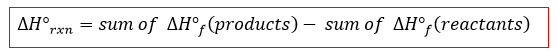# Problem: The following reaction can be used to prepare samples of metals. Determine the enthalpy change under standard state condition.(b) SnO(s) + CO(g) ⟶ Sn(s) + CO2(g)

###### FREE Expert Solution

Recall that for standard state conditions, the enthalpy change, ∆H°rxn can be solved using the equation:To solve for enthalpy change, we need to do these steps:

• Check if the chemical equation is balanced
• Find standard enthalpy of formation for the products and reactants
97% (20 ratings)###### Problem Details

The following reaction can be used to prepare samples of metals. Determine the enthalpy change under standard state condition.

(b) SnO(s) + CO(g) ⟶ Sn(s) + CO2(g)